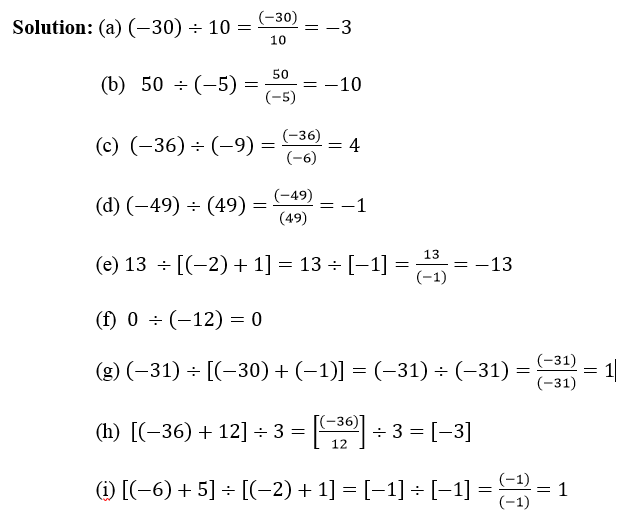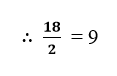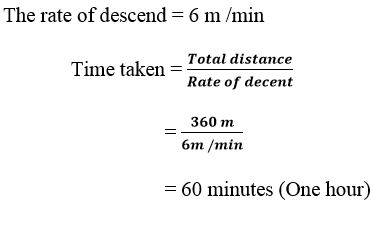## 1.4 DIVISION OF INTEGERS:

Lesson Progress
0% Complete

1.4   DIVISION OF INTEGERS:

If a and b are two  integers such that b ≠ 0 then a ÷ b may or may not be an integer.

If a is an integer and a ≠ 0 then a ÷ −a = −1.

If a is an integer and a ≠ 0 then a ÷ 1 = a .

If a is an integer and a ≠ 0 then 0 ÷ a = 0 .

EXERCISE 1.4

Evaluate each of the following:

a) (–30) ÷ 10                                     b) 50 ÷ (–5)

c) (–36) ÷ (–9)                                 d) (–49) ÷ (49)

e) 13 ÷ [(–2) + 1]                             f) 0 ÷ (–12)

g) (–31) ÷ [(–30) + (–1)]              h) [(–36) ÷ 12] ÷ 3

i) [(–6) + 5] ÷ [(–12) + 1]2) Verify that a÷ (b + c) ≠ (a ÷ b) + (a ÷ c) for each of the following values of a, b and c.

a) a = 12,    b= –4,   c = 2

b) a = –10,   b = 1,   c= 1

Solution: Given that a = 12,    b = –4  and  c = 2

L.H.S = a ÷ (b + c)   = 12 ÷ (–4 + 2)

= 12 ÷ (–2) = –6

R.H.S = (a ÷ b) + (a ÷ c) =  [12 ÷ (–4)] + (12 ÷ 2)

=  (–3) + 6 = 3

∴ –6  ≠  3

∴      L.H.S  ≠  R.H.S

Hence, a ÷ (b ÷ c) ≠ (a ÷ b) +  (a ÷ c)

b) Given that a = –10,   b = 1,   c= 1

L.H.S = a ÷ (b + c) = (–10) ÷ (1 + 1)

= (–10) ÷ 2 = –5

R.H.S = (a ÷ b) + (a ÷ c) = [(–10) ÷ 1] + [(–10) ÷ 1]

= (–10) + (–10) = –20

∴ –5  ≠ –20

∴  L.H.S ≠ R.H.S

Hence, a ÷ (b ÷ c) ≠ (a ÷ b) + (a ÷ c)

3) Fill in the blanks:

a) 369 ÷ ____ = 369                                b) (−75) ÷ ___ = −1

c) (−206) ÷ ____ = 1                               d) −87 ÷ _____ = 87

e) ____ ÷ = −87                                       f) ____÷ 48 = −1

g) 20 ÷ ____ = −2                                   h) ____ ÷ 4 = −3

Solution:

a) ∴ a ÷ 1  = a                                                  ∴ 369 ÷ 1 = 369

b) ∴ (−a) ÷ a = −1                                          ∴ (−75) ÷ 75 = −1

c) ∴  (−a) ÷ −a = 1                                         ∴ (−206) ÷ (−206) = 1

d) ∴ (−a) ÷ (−1) = a                                      ∴ (−87) ÷ (−1) = 87

e) ∴  (−a) ÷ a = −a                                        ∴ (−87) ÷ 1 = − 87

f) ∴  (−a) ÷ a = −1                                         ∴ (−48) ÷ 48  = −1

g) ∴ 20 ÷ 10 = 2                                             ∴ 20 ÷ (−10) = −2

h) ∴ 12 ÷ 4 = 3                                               ∴  (−12) ÷ 4 = −3

4) Write five pairs of integers (a, b) such that a ÷ b = –3. One such pair is  because 6 ÷ (–2) = (–3)

Solution:

i)  Since, 3 ÷ 1 = 3

∴ 3 ÷ (–1) = –3

Comparing it with a ÷ b = –3 we have a = 3 and b = (–1)

∴ The required pair of integers = (3, –1)

ii) Since, 3 ÷ 1 = 3

∴ (–3) ÷ 1 = –3

Comparing it with a ÷ b = –3 we have a = 9 and b = 1

∴ The required pair of integers = (–3, 1)

iii)  Since, 9 ÷ 3 = 3

∴ 9 ÷ (–3) = –3

Comparing it with a ÷ b = –3 we have a =9 and b = –3

∴ The required pair of integers = (9, –3)

iv) Since, 12 ÷ 4 = 3

∴ (–12) ÷ 4 =  –3

Comparing it with a ÷ b = –3 we have a = –12 and b = 4

∴ The required pair of integers = [–12, 4]

v) Since, 12 ÷ 4 = 3

∴ 12 ÷ (–4) = –3

Comparing it with a ÷ b = –3 we have a = 12 and b = –4

∴ The required pair of integers = [12, (–4)]

5. The temperature at 12 noon was 10oC above zero. If it decreases at the rate of 2oC per hour until midnight, at what time would the temperature be 8°C below zero? What would be the temperature at mid-night?

Solution:-

Temperature at 12 noon = + 10०C

Rate of change in temperature = – 2oC per hour

Number of hours from 12 noon to mid – night = 12

∴ Change in temperature in 12 hours = 12० x (–2) = –24०C

∴ Temperature at mid-night (i.e. 12 hours after 12 noon) = +10०C + (24०C)

= – 14oC

Thus, temperature at mid- night = – 14०C

Now temperature difference between + 10०C and – 8 =  + 10०C – (–8०C)

= 18०C∴ Temperature change of 18०C will take place in 9 hours from 12 noon.

Time after 9 hours from 12 noon = 9 p.m.

Thus, the temperature 8०C below 0० (8०C) would be at 9 p.m.

6. In a class test (+ 3) marks are given for every correct answer and (–2) marks are given for every incorrect answer and no marks for not attempting any question.

(i) Radhika scored 20 marks. If she has got 12 correct answers, how many questions has she attempted incorrectly?

(ii) Mohini scores –5 marks in this test, though she has got 7 correct answers. How many questions has she attempted incorrectly?

Solution:-

Marks for every correct answer = + 3

Marks for every incorrect answer = –2

(i)  Total marks scored by Radhika = 20

Marks scored for correct answers = 12 × 3 = 36

∴ Marks given for incorrect answers = 20 – 36 = – 16

∴ Number of incorrect answers = (–16) ÷ (–2) = 8

Thus, Radhika attempted 8 questions incorrectly.

(ii)  Marks obtained by Mohini = –5

Marks obtained for 7 correct answers = 7 × 3 = 21

∴ Marks obtained for incorrect answers = – 5 – 21 = – 26

∴ Number of incorrect answers = (–26) ÷ (–2) = 13

Thus, Mohini attempted 13 questions incorrectly.

7. An elevator descends into a mine shaft at the rate of 6 m/min. If the descent starts from 10 m above the ground level, how long will it take to reach – 350 m.

Solution:-

Present position of the elevator is at 10 m above the ground level.

Distanced to be moved by the elevator below the ground level = 350 m

∴ Total distance to be moved by the elevator = 350 m + 10 m

= 360 m